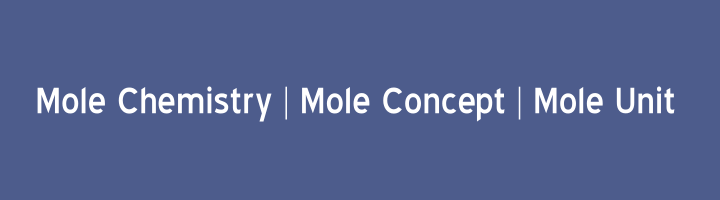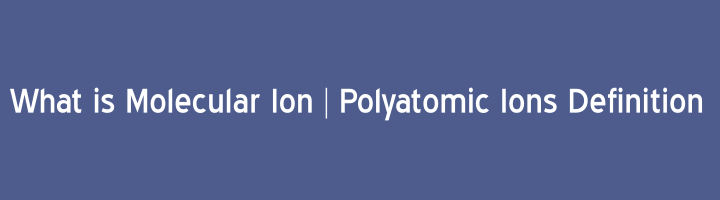The number of atoms, molecules, formula units or ions in one gram atom, one gram molecule, one gram formula unit or one gram ion, respectively is called Avogadro’s number. OR The number of atoms, molecules, formula units or ions in one mole of the substance […]## Mole Chemistry | Mole Concept | Mole Unit

Below are some important points to get clear mole concept, definition of mole chemistry and mole units. Mole can be define as, The atomic mass, molecular mass, ionic mass or formula mass of a substance expressed in grams is called mole of that substance. OR […]## What is Molecular Ion | Polyatomic Ions Definition

“When a molecule loss or gain one or more electrons, is called Molecular Ion or Polyatomic ion.” Examples of Polyatomic ions:                 CH4+, CO+, N2+ etc. Formation of Molecular Ion:                                 Cationic molecular ions are more abundant than anionic Molecular ions. These ions can be […]